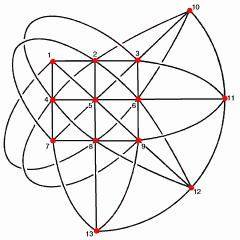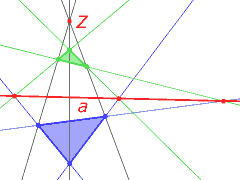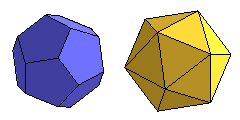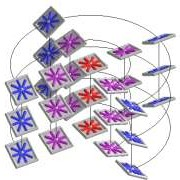# Hans Havlicek: Projective Geometry

This series of lectures for students of Descriptive Geometry (teacher's programme) gives an introduction to projective, affine and Euclidean geometry.

## Contents

The subsequent list refers to my lectures in the years 2001-2003 according to the old curriculum.Projective plane with 13 pointsAxiom of Desargues

## 1. Affine and Projective Planes

• Axioms of affine and projective planes
• Connection between affine and projective planes
• Principle of duality

## 2. Projectivities and Collineations

• Perspectivities
• Projectivities
• Axioms of Desargues and Pappos
• Hessenberg's theorem
• Perspective and projective collineations
• Fano's axiom

## 3. Affinities

• Perspectivities in affine planes
• Perspective and projective affinities

## 4. Conics

• Steiner's definition
• Pascal's theorem
• Projectivities on conics
• External and internal points
• Nuclei
• Polarity with respect to a conic
• Higher-order contact of conics
• Pencils of conics
• Conics in affine planes

## 5. Affine and Projective Spaces

• Axioms of affine and projective spaces
• Connection between affine and projective spaces

## 6. Properties of Projective Spaces

• Lattice of subspaces
• Dimension formula of Grassmann

## 7. Properties of Affine Spaces

• Parallel subspaces
• Hyperplane at infinity
• Affine geometry in projective terms

## 8. The Dual of a Projective Space

• Pencils of hyperplanes
• Dual space
• Principle of duality
• Bidual of a projective space

## 9. Projectivities and Collineations of Projective Spaces

• Projectivities
• Perspective and projective collineations
• Transitivity properties of the projective groupDual polyhedra

## 10. Correlations

• Correlations
• Polarities
• Conjugate points
• Auto-polar simplices
• absolute points
• Types of polarities

• Polarity with respect to a quadric
• Common points of two conics
• ReguliGeneral linear complex of lines

## 12. Null Polarities and Line Geometry in 3-Spaces

• Null polarities in 3-spaces
• General and special linear complexes of lines
• Hyperbolic, parabolic, and elliptic congruences of lines

## 13. Twisted Cubics

• Seydewitz's definition
• Chords
• tangents and osculating planes
• Polarity with respect to a twisted cubic
• Cubic developables
• Axes## 14. Projective and Affine Spaces over Vector Spaces

• Projective spaces over vector spaces
• Fundamental theorem of projective geometry
• Affine spaces over vector spaces
• Fundamental theorem of affine geometry
• Representation of collineations and affinities in terms of semilinear mappings
• Projective and affine coordinates
• Cross ratios and affine ratios

## 15. Real and Complex Projective Spaces

• Complexification of real spaces
• Complex conjugate elements

## 16. Euclidean Spaces

• Orthogonality in terms of an absolute polarity
• Similarities
• Spheres
• Direct and opposite congruence transformations
• Euclidean spaces over vector spaces
• Angles and lengths
• Axes and umbilical points of quadrics
• Foci of conics